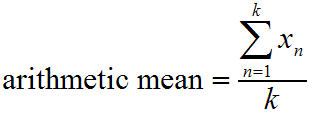# The Arithmetic Mean

An arithmetic mean is a fancy term for what most people call an "average." When someone says the average of 10 and 20 is 15, they are referring to the arithmetic mean. The simplest formula for a mean is the following: Add up all the numbers you want to average, and then divide by the number of items you just added.

For example, if you want to average 10, 20, and 27, first add them together to get 10+20+27= 57. Then divide by 3 because we have three values, and we get an arithmetic mean (average) of 19.

Want a formal, mathematical expression of the arithmetic mean?That's just a fancy way to say "the sum of k different numbers divided by k."

Check out a few examples of the arithmetic mean to make sure you understand:

Example:

Find the arithmetic mean (average) of the following numbers: 9, 3, 7, 3, 8, 10, and 2.

Solution:

Add up all the numbers. Then divide by 7 because there are 7 different numbers.

$$\frac{9+3+7+3+8+10+2}{7} = \frac{42}{7} = 6$$

Example:

Find the arithmetic mean of -4, 3, 18, 0, 0, and -10.

Solution:

Add the numbers. Divide by 6 because there are 6 numbers.

The answer is $$\frac{7}{6}$$, or approximately 1.167

### Summary

Two variables vary directly if their ratio always remains the same; when one doubles so does the other.